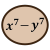Games
Problems
Go Pro!

# Polynomials

Reference > Mathematics > AlgebraPolynomials - covering some basic terms and usage informationPolynomials - more definitions and examples of polynomials and polynomial equationsHow to evaluate a polynomial for a given value of the variableFundamental theorem of Algebra, finding the zeroes of a polynomialTying coefficients to roots in polynomial equationsExpanding what we learned about coefficients and roots to higher degree polynomialsThe sum of the roots taken n at a time correlates to the coefficients of the polynomialUsing synthetic division to find the quotient when a polynomial is divided by a binomialHow to find rational roots of a polynomial equation using the rational root theoremIrrational roots and complex roots, conjugates, and polynomial equations# Blogs on This SiteReviews and book lists - books we love!The site administrator fields questions from visitors.Like us on Facebook to get updates about new resources